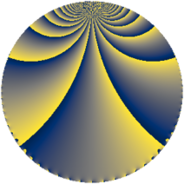Properties

 Label 1050.3.kLevel $1050$ Weight $3$ Character orbit 1050.k Rep. character $\chi_{1050}(293,\cdot)$ Character field $\Q(\zeta_{4})$ Dimension $192$ Sturm bound $720$

Related objects

Defining parameters

 Level: $$N$$ $$=$$ $$1050 = 2 \cdot 3 \cdot 5^{2} \cdot 7$$ Weight: $$k$$ $$=$$ $$3$$ Character orbit: $$[\chi]$$ $$=$$ 1050.k (of order $$4$$ and degree $$2$$) Character conductor: $$\operatorname{cond}(\chi)$$ $$=$$ $$105$$ Character field: $$\Q(i)$$ Sturm bound: $$720$$

Dimensions

The following table gives the dimensions of various subspaces of $$M_{3}(1050, [\chi])$$.

Total New Old
Modular forms 1008 192 816
Cusp forms 912 192 720
Eisenstein series 96 0 96

Trace form

 $$192 q + 8 q^{7} + O(q^{10})$$ $$192 q + 8 q^{7} - 768 q^{16} - 32 q^{18} - 48 q^{21} + 80 q^{22} + 16 q^{28} + 160 q^{36} - 64 q^{37} + 64 q^{42} + 64 q^{43} + 192 q^{46} - 336 q^{51} - 584 q^{57} - 224 q^{58} - 240 q^{63} - 64 q^{67} + 64 q^{72} + 128 q^{78} - 304 q^{81} + 160 q^{88} + 88 q^{91} + 840 q^{93} + O(q^{100})$$

Decomposition of $$S_{3}^{\mathrm{new}}(1050, [\chi])$$ into newform subspaces

The newforms in this space have not yet been added to the LMFDB.

Decomposition of $$S_{3}^{\mathrm{old}}(1050, [\chi])$$ into lower level spaces

$$S_{3}^{\mathrm{old}}(1050, [\chi]) \cong$$ $$S_{3}^{\mathrm{new}}(105, [\chi])$$$$^{\oplus 4}$$$$\oplus$$$$S_{3}^{\mathrm{new}}(210, [\chi])$$$$^{\oplus 2}$$$$\oplus$$$$S_{3}^{\mathrm{new}}(525, [\chi])$$$$^{\oplus 2}$$